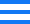首页 > 企业 > 企业要闻

## 陕西省属国企领导年薪一览表

80万元以上的：

陕西煤业化工集团董事长 杨照乾 72.09+10.63+0=82.72万元

陕西投资集团董事长 袁小宁 66.71+15.46+0=82.17万元

延长石油集团董事长 杨悦 70.68+9.9+0=80.58万元

70万-80万元的：

长安银行董事长 毛亚社 62.39+16.14+0=78.53万元

陕西地电董事长 刘斌 63.29+13.56+0=76.85万元

陕西有色集团董事长 马宝平 63.70+12.45+0=76.15万元

陕西建工集团董事长 张义光 65.1263+9.3639+0=74.4902万元

秦农银行行长 郝光耀 55.63+18.53+0=74.16万元

60万-70万元的：

陕西省农村信用联社主任 张全明 45.37+21.01+0=66.38万元

陕西旅游集团董事长 周冰 52.89+11.43+0=64.32万元

陕西地建集团执行董事、总经理韩霁昌 55.5450+7.3540+0=62.8990万元

永安财险董事长 陶光强 52.93+9.43=0=62.36万元

陕西粮农集团董事长 王东峰 50.04+10.03+0=60.07万元

50万-60万元的：

秦川集团董事长 龙兴元 税前总报酬58.55万元

陕西高速集团董事长 靳宏利 46.2875+9.55+0=55.8375万元

陕西电子信息集团董事长 燕林豹 43.80+11.31+0=55.11万元

引汉济渭公司党委书记、执行董事 杜小洲 44.39+10.85=0=55.24万元

陕西交通建设集团董事长 黄会奇 44.3+9.21+0=53.51万元

陕西环保产业集团董事长 熊良虎 42.69+10.43+0=53.12万元

陕西省外经贸实业集团董事长 肖玉龙 43.6+9.27+0=52.87万元

陕西地矿集团董事长 赵廷周 47.47+4.68+0=52.15万元

陕西水务集团董事长 王瑜 41.62+10.28+0=51.9万元

陕西铁路集团董事长 乔怀玉 41.78+9.66+0=51.44万元

陕药董事长 翟日强 45.9665+5.1985+0=51.1650万元

陕西燃气集团董事长 郝晓晨 38.40+12.39+0=50.79万元

40万-50万元的：

西部机场集团董事长 王海鹏 39.86+8.75+0=48.61万元

中陕核工业集团董事长 张斌成 45.31+2.51+0=47.82万元

陕西省物流集团董事长 郑忠堂 35.12+9.0888+0=44.2088万元

陕西金融控股集团董事长 刘红旗 36.33+7.65+0=43.98万元

东庄水利枢纽工程建设公司董事长 肖宏武 32.35+8.98+0=41.33万元

陕西果业集团董事长 高建国 34.15+8.241+0=42.391万元

40万元以下的：

陕西林业集团董事长 方有为 11.48+5.80+0=17.28万元